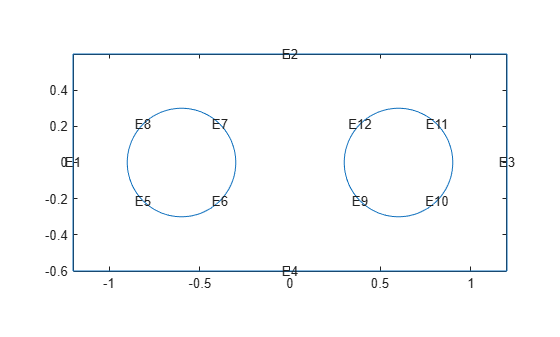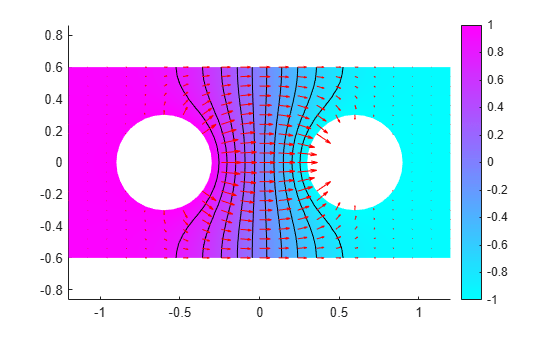# Current Density Between Two Metallic Conductors

This example shows how to find the electric potential and the components of the current density between two circular metallic conductors. Two metallic conductors are placed on a brine-soaked blotting paper that serves as a plane, thin conductor. The physical model for this problem is DC conduction. The boundary conditions are:

• The electric potential V = 1 on the left circular conductor

• The electric potential V = –1 on the right circular conductor

• No surface current on the outer boundaries of the plane conductor

First, create a geometry consisting of a rectangle and two circles. Start by defining a rectangle and two circles.

```R1 = [3;4 -1.2;-1.2;1.2;1.2 -0.6;0.6;0.6;-0.6]; C1 = [1;-0.6;0;0.3]; C2 = [1;0.6;0;0.3];```

Append extra zeros to the circles so they have the same number of rows as the rectangle.

```C1 = [C1;zeros(length(R1) - length(C1),1)]; C2 = [C2;zeros(length(R1) - length(C2),1)];```

Combine the shapes into one matrix.

`gd = [R1,C1,C2];`

Create names for the rectangle and the circles, and specify the formula to create the geometry.

```ns = char('R1','C1','C2'); ns = ns'; sf = 'R1 - (C1 + C2)'; g = decsg(gd,sf,ns);```

Create an electromagnetic model for DC conduction analysis.

`model = createpde("electromagnetic","conduction");`

Include the geometry in the model, and plot it with the edge labels.

```geometryFromEdges(model,g); pdegplot(model,"EdgeLabels","on")```Specify the conductivity of the material as σ = 1.

`electromagneticProperties(model,"Conductivity",1);`

Specify the electric potential values on the left and right circular conductors.

```electromagneticBC(model,"Edge",5:8,"Voltage",1); electromagneticBC(model,"Edge",9:12,"Voltage",-1);```

Specify the zero surface current density on the outer boundaries.

`electromagneticBC(model,"Edge",1:4,"SurfaceCurrentDensity",0);`

Generate the mesh.

`generateMesh(model);`

Solve the model.

`R = solve(model)`
```R = ConductionResults with properties: ElectricPotential: [1021x1 double] ElectricField: [1x1 FEStruct] CurrentDensity: [1x1 FEStruct] Mesh: [1x1 FEMesh] ```

Plot the resulting electric potential and current density, and display the equipotential lines. The current flows from the conductor with a positive potential to the conductor with a negative potential. The conductivity σ is isotropic, and the equipotential lines are orthogonal to the current lines.

```pdeplot(model,"XYData",R.ElectricPotential, ... "Contour","on", ... "FlowData",[R.CurrentDensity.Jx,R.CurrentDensity.Jy]) axis equal```